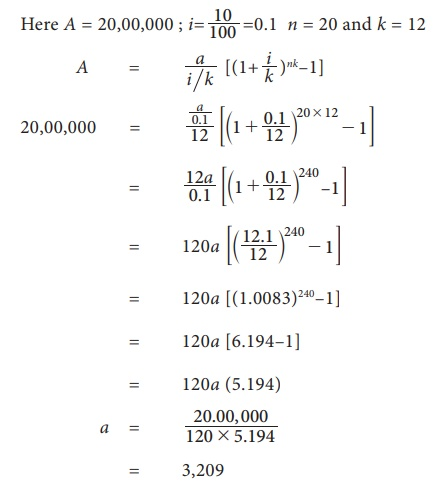Home | | Business Maths 11th std | Example Problems for Annuities

# Example Problems for Annuities

Example Problems and Questions for Annuities

Example 7.1

A person pays 64,000 per annum for 12 years at the rate of 10% per year. Find the annuity [(1.1)12=3.3184]

Solution :Example 7.2

What amount should be deposited annually so that after 16 years a person receives 1,67,160 if the interest rate is 15% [(1.15)16=9.358]

Solution :Example 7.3

The age of the daughter is 2 years. Her father wants to get 20,00,000 when his ward becomes 22 years He opens an account with a bank at 10% rate of compound interest. What amount should he deposit at the end of every month in this recurring account? [(1.0083)240=6.194].

Solution :3,209 is to be deposited at the end of every month.

Example 7.4

A person deposits 4,000 in the beginning of every year. If the rate of compound interest is 14% then ,find the amount after 10 years . [(1.14)10=3.707]

Solution :Example 7.5

A person purchases a machine on 1st January 2009 and agrees to pay 10 installments each of 12,000 at the end of every year inclusive of compound rate of 15%. Find the present value of the machine. [(1.15)10=4.016].

Solution :Example 7.6

A photographer purchases a camera on installments. He has to pay 7 annual installments each of 36,000 right from the date of purchase. If the rate of compound interest is 16% then find the cost price (present value) of the camera. [(1.16)7=2.2828]

Solution :Example 7.7

A person has taken a loan of 7,00,000 at 16% rate of interest from a finance company. If the repayment period is of 15 years then find the installment he has to pay at the beginning of each month. [(1.0133)180=9.772]

Solution :Example 7.8

The chairman of a society wishes to award a gold medal to a student getting highest marks in Business Mathematics. If this medal costs 9,000 every year and the rate of compound interest is 15% what amount is to be deposited now.

Solution :

Here a = 9,000 and i = 0.15Therefore the amount to be deposited is 60,000.

Example 7.9

A limited company wants to create a fund to help their employees in critical circumstances. The estimated expenses per month is 18,000.Find the amount to be deposited by the company if the rate of compound interest is 15%.

Solution :

Here a = 18,000 ; i = 0.15 and k = 12.Therefore the amount to be deposited is 14,40,000.

Example 7.10

Find the half yearly rate of interest, to get a perpetuity of 675 for every half yearly from the present value of 30,000/

Solution :

Here P=30,000 ; a = 675 ; k = 2, i = ?Exercise 7.1

1. Find the amount of an ordinary annuity of 3,200 per annum for 12 years at the rate of interest of 10% per year. [(1.1)12 = 3.3184]

2. If the payment of 2,000 is made at the end of every quarter for 10 years at the rate of 8% per year, then find the amount of annuity. [(1.02)40=2.2080 ]

3. Find the amount of an ordinary annuity of 12 monthly payments of 1, 500 that earns interest at 12% per annum compounded monthly. [(1.01)12 = 1.1262 ]

4. A bank pays 8% per annum interest compounded quarterly. Find the equal deposits to be made at the end of each quarter for 10 years to have 30,200 ? [(1.02)40 =2.2080]

5. A person deposits 2,000 from his salary towards his contributory pension scheme. The same amount is credited by his employer also. If 8% rate of compound interest is paid, then find the maturity amount at end of 20 years of service. [(1.0067)240 = 3.3266 ]

6. Find the present value of 2,000 per annum for 14 years at the rate of interest of 10% per annum. [ (1.1)–14= 0.2632]

7. Find the present value of an annuity of 900 payable at the end of 6 months for 6 years. The money compounded at 8% per annum. [(1.04)–12=0.6252 ]

8. Find the amount at the end of 12 years of an annuity of 5,000 payable at the beginning of each year, if the money is compounded at 10% per annum.

9. What is the present value of an annuity due of 1,500 for 16 years at 8% per annum? [ (1.08)15 = 3.1696 ]

10. What is the amount of perpetual annuity of 50 at 5% compound interest per year?

Tags : Financial Mathematics , 11th Business Mathematics and Statistics(EMS) : Chapter 7 : Financial Mathematics
Study Material, Lecturing Notes, Assignment, Reference, Wiki description explanation, brief detail
11th Business Mathematics and Statistics(EMS) : Chapter 7 : Financial Mathematics : Example Problems for Annuities | Financial Mathematics